Mobile QR Code1. (School of Electronic and Electrical Engineering, Kyungpook National University, Daegu, 702-201, Korea )
2. (Korea Multi-purpose Accelerator Complex, Korea Atomic Energy Research Institute, Gyeongju 38180, Korea )
3. (Power Semiconductor Research Center, Korea Electrotechnology Research Institute, Changwon 51543, Korea)
4. (4DB HiTek, 90, Sudo-ro, Bucheon-si, Gyeonggi-do, Korea)

GaN, AlGaN, dual gate dielectric, silicon nitride (Si$_{3}$N$_{4}$), titanium dioxide (TiO$_{2}$), normally-off, recessed gate technique

## I. INTRODUCTION

With the recent advances in technology, the development of applications requiring high power and efficiency, such as computers, electric vehicles, solar power, and smart grids is emerging. Electric vehicles require high-performance power semiconductor devices. Gallium nitride (GaN) has attracted great attention for applications in power electronics due to its wide bandgap, high critical electric field, and thermal resistance [1-5].

GaN-based high electron mobility transistors (HEMT) have high breakdown voltage due to the material properties of GaN. In addition, since the on-resistance is reduced via the two-dimensional electron gas (2DEG) with high electron mobility caused by the AlGaN/GaN junction as a channel, it is suitable for high-frequency and high-power semiconductors. However, in the general AlGaN/GaN HEMT, the 2DEG layer is used as a channel to have a negative threshold voltage ($\textit{V}$$_{\mathrm{th}}), so power consumption is high. Therefore, it is very important for the design to have a positive \textit{V}$$_{\mathrm{th}}$ to reduce the power loss .

Recently, many technologies for realizing normally-off HEMT through various methods such as gate injection transistor (GIT) [7-9], fluorine plasma treatment [10-12], and recessed gate metal insulator semiconductor (MIS) structure [13-17] have been studied. GIT is difficult to grow p-GaN and the output current is relatively low as compared to other structures. Additionally, when GaN is grown by metal organic chemical vapor deposition (MOCVD), it becomes n-GaN, so that it is difficult to dope the p-type to grow p-GaN. Fluorine plasma treatment is unstable at high temperatures. Conversely, the recessed gate MIS structure has a relatively simple process and high output current compared to the GIT structure. In addition, since there is an insulator under the gate, it is possible to effectively reduce the gate leakage current [18-23].

In this paper, a dual gate insulator is adopted to prevent the increase in the gate leakage current and the off-current and to improve the on-current for general recessed gate AlGaN/GaN MOSFETs. Therefore, Si$_{3}$N$_{4}$ (${\upvarepsilon}$ = 8.9) with relatively better interfacial properties than TiO$_{2}$ (${\upvarepsilon}$ = 80) was first deposited on GaN, and high-k TiO$_{2}$ was deposited on Si$_{3}$N$_{4}$ to fabricate a device. We fabricated two types of dual gate dielectric-based devices with different thicknesses of Si$_{3}$N$_{4}$ and TiO$_{2}$ (Si$_{3}$N$_{4}$/TiO$_{2}$ = 10/20 nm and 20/10 nm) and Si$_{3}$N$_{4}$ single dielectric-base device with the same process. We compared the electric characteristics of the three devices.

## II. STRUCTURE AND FABRICATION

Fig. 1(a) and (b) show the schematic cross-sectional view of the single gate dielectric- and dual gate dielectric-based devices, respectively. Fig. 1(c) shows the optical microscope image of the fabricated recessed gate AlGaN/GaN MOSFET. The dual gate dielectric-based device consists of Si$_{3}$N$_{4}$ gate dielectric at the bottom and TiO$_{2}$ gate dielectric at the top. Si$_{3}$N$_{4}$ instead of TiO$_{2}$ is chosen as the material to be deposited directly on GaN as the gate leakage current increases when high-k TiO$_{2}$ is directly bonded to GaN. Later, when TiO$_{2}$ is stacked and used as a dual gate dielectric, the increased oxide capacitance can lead to a high on-state current without significant change in the gate leakage current due to the Si$_{3}$N$_{4}$/GaN junction.

Fig. 2 shows the process flow of the recessed gate AlGaN/GaN MOSFET with Si$_{3}$N$_{4}$/TiO$_{2}$ stacked dual dielectric. The fabricated device was an epitaxial growth of GaN and AlGaN layers using MOCVD on a sapphire substrate. The thicknesses of sapphire substrate, GaN buffer, GaN channel, and AlGaN layers are 430 ${μ}$m, 2.1 ${μ}$m, 180 nm, and 25 nm, respectively. The Al composition in the AlGaN layer was 21%. The sheet carrier density and the electron mobility obtained by hall measurements were 8 ${\times}$ 10$^{12}$cm$^{-2}$ and 1200 cm/V${\cdot}$s, respectively. To physically insulate each device, 380 nm depth mesa insulation was performed by Cl$_{2}$-based inductively coupled plasma-reactive ion etcher (ICP-RIE). After the mesa process, a 50 nm-thick Si$_{3}$N$_{4}$ layer was deposited via plasma enhanced chemical vapor deposition (PECVD) to be used as a hard mask in the recessed gate process. In the gate recess etching process, a 25 nm-thick AlGaN layer was etched by Cl$_{2}$-based ICP-RIE. Then, the Si$_{3}$N$_{4}$ layer used as the gate dielectric was deposited via PECVD. Subsequently, TiO$_{2}$ layer used as the gate dielectric was deposited by atomic layer deposition. Before depositing the ohmic contact metal, BOE solution was used to open the oxide at the location to enter the source and drain metals. Next, the material to be used as the ohmic contact metal of the source and drain consisting of Ti/Al/Ni/Au (25/160/40/100 nm), was deposited using an electron-beam (E-beam) evaporator. To form an ohmic contact, annealing was performed at 800$^{\circ}$C for 30 s in the nitrogen (N$_{2}$) atmosphere. Finally, a gate metal composed of Ni/Au (40/100 nm) material was deposited using an E-beam evaporator.

##### Fig. 1. The schematic cross-sections of the recessed gate AlGaN/GaN MOSFET with (a) the single gate dielectric, (b) dual gate dielectric, (c) The optical microscope image of the fabricated the recessed gate AlGaN/GaN MOSFET.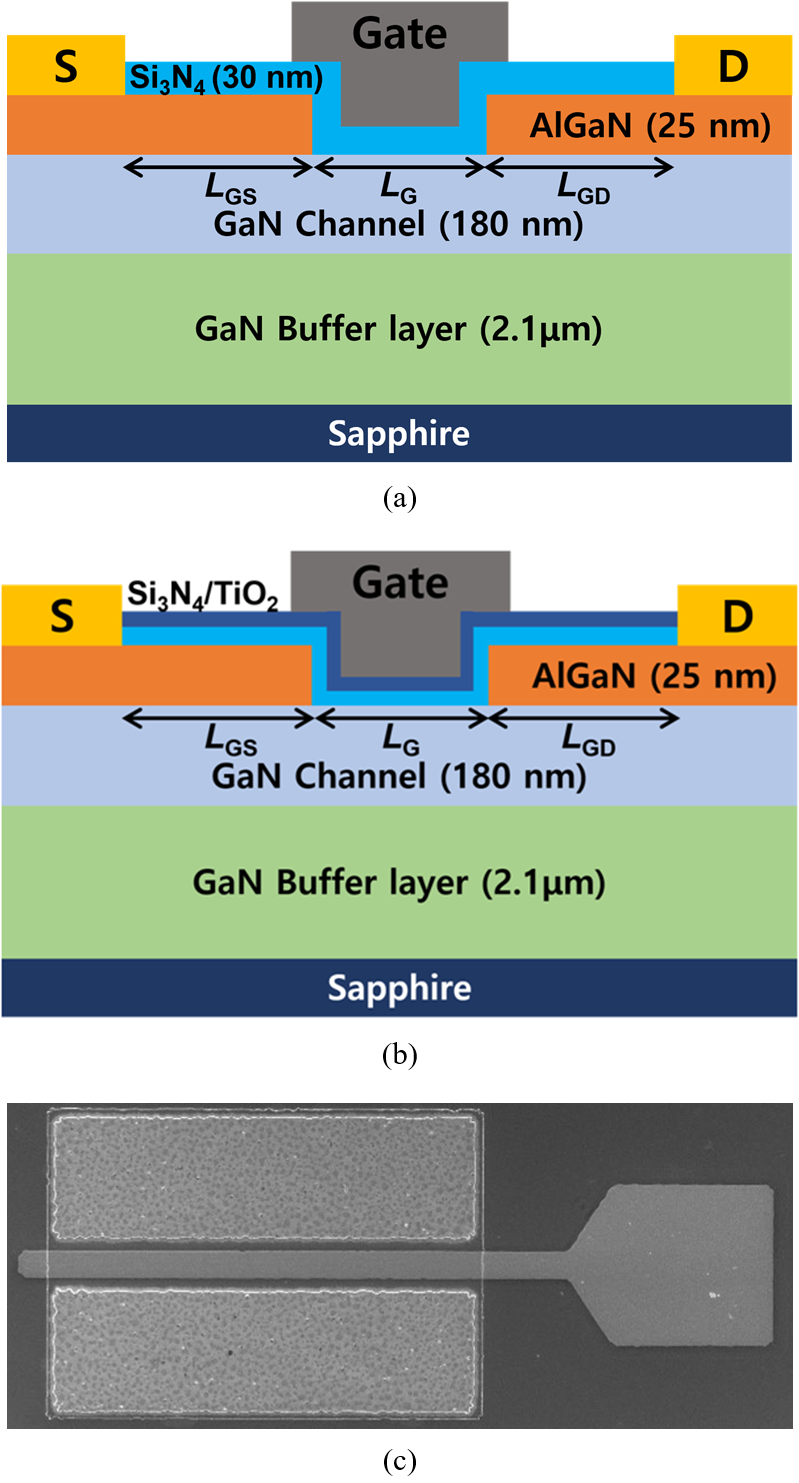##### Fig. 2. Process flow of the recessed gate AlGaN/GaN MOSFET with Si$_{3}$N$_{4}$/TiO$_{2}$ stacked dual dielectric.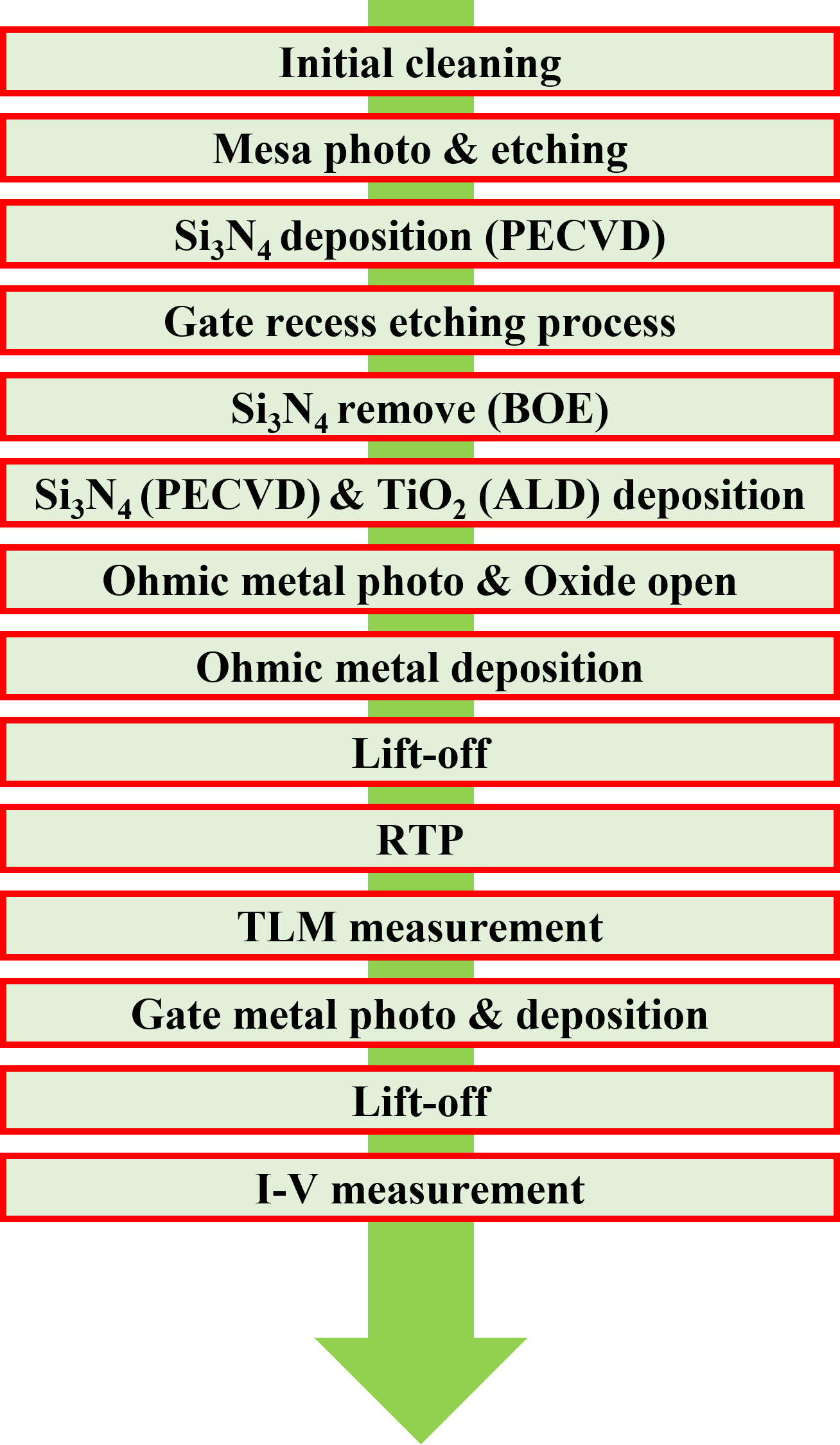## III. RESULTS AND DISCUSSION

Fig. 3(a) and (b) show the transfer curve of the single gate dielectric and dual gate dielectric-based devices. The on- state drain current ($\textit{I}$$_{\mathrm{D,max}}) is defined at \textit{V}$$_{\mathrm{GS}}$ = 10 V and $\textit{V}$$_{\mathrm{DS}} = 10 V. \textit{V}$$_{\mathrm{th}}$ is obtained by linear extrapolation. A dual dielectric-based device has higher on-current and transconductance ($\textit{g}$$_{\mathrm{m}}) than a single dielectric-based device. Among the two types, the dual gate dielectric (Si_{3}N_{4}/TiO_{2} = 10/20 nm)-based device exhibited the highest current characteristics, with the \textit{V}$$_{\mathrm{th}}$ of 1.81 V, $\textit{I}$$_{\mathrm{D,max}} of 7.95 mA, \textit{g}$$_{\mathrm{m}}$ of 1.12 mS, and subthreshold swing ($\textit{SS}$) of 229 mV/dec. The measured electrical characteristics of the fabricated devices are summarized in Table 1. The current characteristics were improved for the dual gate dielectric because of higher capacitance than the single Si$_{3}$N$_{4}$ gate dielectric. The formula for calculating the capacitance is as follows:

##### (1)
$$\mathrm{C}_{\mathrm{TiO}_{2}}=\frac{\mathcal{E}_{\mathrm{TiO}_{2}} \cdot \mathcal{E}_{0}}{\mathrm{t}_{\mathrm{TiO}_{2}}}$$
##### (2)
$$\mathrm{C}_{\mathrm{Si}_{3} \mathrm{~N}_{4}}=\frac{\varepsilon_{\mathrm{Si}_{3} \mathrm{~N}_{4}} \cdot \varepsilon_{0}}{\mathrm{t}_{\mathrm{Si}_{3} \mathrm{~N}_{4}}}$$
##### (3)
$$\frac{1}{\mathrm{C}_{\text {total }}}=\frac{1}{\mathrm{C}_{\mathrm{Si}_{3} \mathrm{~N}_{4}}}+\frac{1}{\mathrm{C}_{\mathrm{TiO}_{2}}}$$

where $\varepsilon _{{\mathrm{TiO}_{2}}}$(=80) and $\varepsilon _{{\mathrm{Si}_{3}}{\mathrm{N}_{4}}}$(=8) are the relative dielectric constants of TiO$_{2}$ and Si$_{3}$N$_{4}$, respectively. $\varepsilon _{0}$ is the vacuum permittivity. $\mathrm{t}_{{\mathrm{TiO}_{2}}}$and $\mathrm{t}_{{\mathrm{Si}_{3}}{\mathrm{N}_{4}}}$are the thicknesses of TiO$_{2}$ and Si$_{3}$N$_{4}$, respectively. $\mathrm{C}_{{\mathrm{TiO}_{2}}}$ and $\mathrm{C}_{{\mathrm{Si}_{3}}{\mathrm{N}_{4}}}$ are the capacitances of TiO$_{2}$ and Si$_{3}$N$_{4}$, respectively, and $\mathrm{C}_{\text{total}}$ is the total accumulation capacitance. The calculated capacitances of the single gate dielectric (Si$_{3}$N$_{4}$ = 30 nm), dual gate dielectric (Si$_{3}$N$_{4}$/TiO$_{2}$ = 20/10 nm) and dual gate dielectric (Si$_{3}$N$_{4}$/TiO$_{2}$ = 10/20 nm) were 236 nF/cm$^{2}$, 337 nF/cm$^{2}$, and 590 nF/cm$^{2}$ respectively. In MOSFET, $\textit{I}$$_{\mathrm{D}} is proportional to \textit{C}$$_{\mathrm{ox}}$, so using a dual gate dielectric increases the on-current. Therefore, compared to the single gate dielectric-based device, the $\textit{I}$$_{\mathrm{D,max}} and \textit{g}$$_{\mathrm{m}}$ of the dual gate dielectric-based device were improved by 292% and 195%, respectively.

##### (5)
$D_{\mathrm{it}}=\frac{1}{0.4\mathrm{Aq}}\left(\frac{G_{\mathrm{p}}}{\omega }\right)_{\max }\approx \frac{2.5}{\mathrm{Aq}}\left(\frac{G_{\mathrm{p}}}{\omega }\right)_{\max }$

where A is the area of the proposed device and q is the electronic charge in coulombs. $\textit{D}$$_{\mathrm{it}} of the single gate dielectric (Si_{3}N_{4} = 30 nm), dual gate dielectric (Si_{3}N_{4}/TiO_{2} = 20/10 nm), and dual gate dielectric (Si_{3}N_{4}/TiO_{2} = 10/20 nm)-based device extracted from the Eq. (5) were 2.79 {\times} 10^{13} cm^{-2}$${\cdot}$eV$^{-1}$, 1.37 ${\times}$ 10$^{14}$ cm$^{-2}$${\cdot}eV^{-1}, and 2.53 {\times} 10^{12} cm^{-2}$${\cdot}$eV$^{-1}$, respectively.

Fig. 5(a)-(c) show the pulsed $\textit{I}$${-}$$\textit{V}$ curves of the single gate dielectric and dual gate dielectric-based devices. Pulsed $\textit{I}$${-}$$\textit{V}$ measurement was performed using the curve tracer B1500A instrument. Gate stress bias $\textit{V}$$_{\mathrm{GS}} = {-}2 V was applied to completely turn-off the device. Specific on resistances (\textit{R}$$_{\mathrm{on}}$) of the single-gate dielectric (Si$_{3}$N$_{4}$= 30 nm), dual-gate dielectric (Si$_{3}$N$_{4}$/TiO$_{2}$ = 20/10 nm), and dual-gate dielectric (Si$_{3}$N$_{4}$/TiO$_{2}$ = 10/20 nm)-based devices were 116 ${\omega}$${\cdot}mm, 58 {\omega}$${\cdot}$mm, and 43 ${\omega}$${\cdot}mm, respectively. And the {\Delta}$$\textit{I}$$_{\mathrm{D,max}} was 1.64%, 3.5%, and 2.91%, respectively. The {\Delta}$$\textit{I}$$_{\mathrm{D,max}} of the three devices was low due to the MIS structure which improved the gate lag. \textit{R}$$_{\mathrm{on}}$ was 62% improvement in the dual gate dielectric-based device with a higher capacitance than a single-gate dielectric-based device, so the performance improvement can be expected in a switching device.

Fig. 6 shows the breakdown voltage (BV) characteristics of the single gate dielectric and dual gate dielectric-based devices with an off-state. BV was extracted at $\textit{I}$$_{\mathrm{D}}=1 mA/mm. The BV values of single gate dielectric (Si_{3}N_{4} = 30 nm), dual gate dielectric (Si_{3}N_{4}/TiO_{2} = 20/10 nm), and dual gate dielectric (Si_{3}N_{4}/TiO_{2} = 10/20 nm)-based devices were 572 V, 556 V and 564 V, respectively, with no significant difference because of no change in the structure. Fig. 7 shows the simulated results of the single gate dielectric (Si_{3}N_{4} = 30 nm) and dual gate dielectric (Si_{3}N_{4}/TiO_{2} = 10/20 nm)-based devices at \textit{V}$$_{\mathrm{GS}}$ = ${-}$2.5 V and $\textit{V}$$_{\mathrm{DS}} = 500 V. Fig. 7(a) and (b) show the contour map of the electric field distribution of the single gate dielectric (Si_{3}N_{4} = 30 nm) and dual gate dielectric (Si_{3}N_{4}/TiO_{2} = 10/20 nm)-based devices. Fig. 7(c) shows the electric field distribution along the A{-}A^{\prime} cut line at the peak electric field strength, and the electric fields of single gate dielectric- and dual gate dielectric (Si_{3}N_{4}/TiO_{2} = 10/20 nm)-based devices were almost similar. BV is greatly affected by the peak electric field with a little change in BV because of a small change in the peak electric field. ##### Fig. 3. The transfer curve of single gate dielectric and dual gate dielectric-based device with (a) \textit{g}$$_{\mathrm{m}}$, (b) gate current.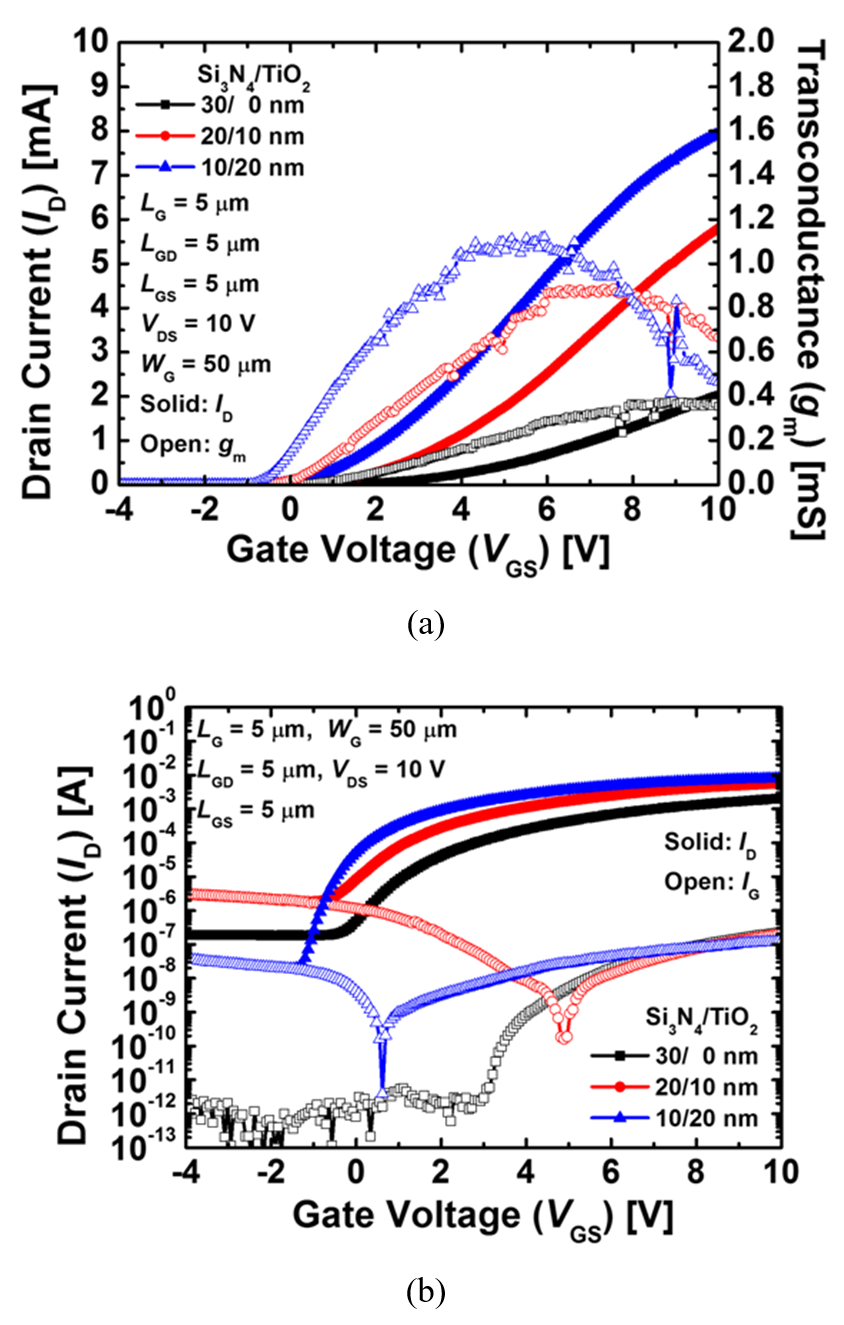##### Fig. 4. (a) The $\textit{C}$${-}$$\textit{V}$ curves, (b) $\textit{G}$$_{\mathrm{p}}/{\omega} vs \textit{V}$$_{\mathrm{gs}}$ characteristics of the single gate dielectric and dual gate dielectric-based devices.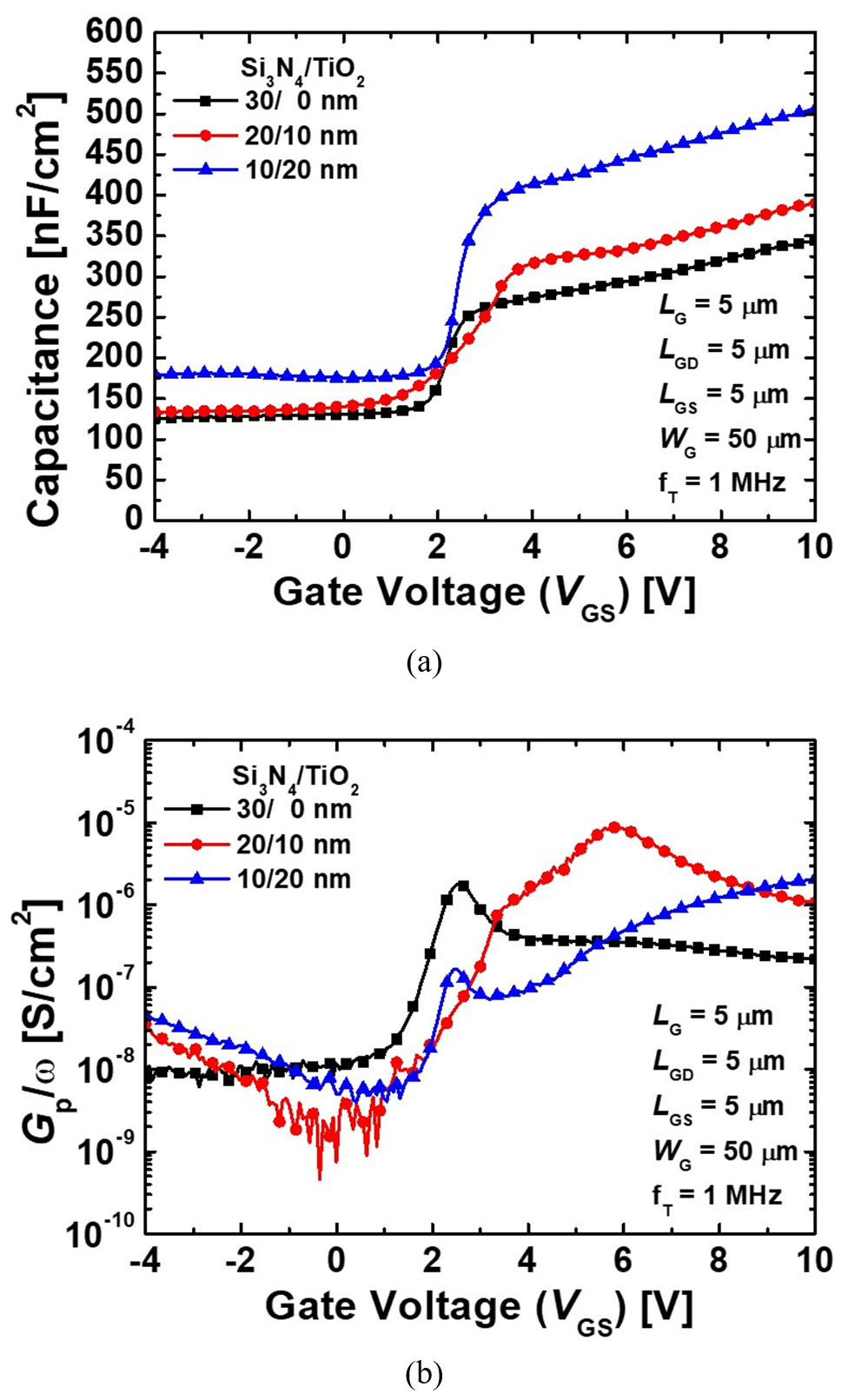## IV. CONCLUSION

This work was supported by the National Research Foundation of Korea (NRF) grant funded by the Korea government (MSIT) (No. NRF-2020R1A2C1005087). This study was supported by the BK21 FOUR project funded by the Ministry of Education, Korea (4199990113966). This research was supported by Basic Science Research Program through the National Research Foundation of Korea (NRF) funded by the Ministry of Education (NRF-2021R1A6A3A13039927). This research was supported by National R&D Program through the National Research Foundation of Korea (NRF) funded by Ministry of Science and ICT (2021M3F3A2A03017764). This investigation was financially supported by Semiconductor Industry Collaborative Project between Kyungpook National University and Samsung Electronics Co. Ltd. The EDA tool was supported by the IC Design Education Center (IDEC), Korea.

## ACKNOWLEDGMENTS

This work was supported by the National Research Foundation of Korea (NRF) grant funded by the Korea government (MSIT) (No. NRF-2020R1A2C1005087). This study was supported by the BK21 FOUR project funded by the Ministry of Education, Korea (4199990113966). This research was supported by Basic Science Research Program through the National Research Foundation of Korea (NRF) funded by the Ministry of Education (NRF-2021R1A6A3A13039927). This research was supported by National R&D Program through the National Research Foundation of Korea (NRF) funded by Ministry of Science and ICT (2021M3F3A2A03017764). This investigation was financially supported by Semiconductor Industry Collaborative Project between Kyungpook National University and Samsung Electronics Co. Ltd. The EDA tool was supported by the IC Design Education Center (IDEC), Korea.

## References

1
Yoon Y. J., Lee J. S., Kim D. S., Lee J. H., Kang I. M., 2020., Gallium Nitride Normally Off MOSFET Using Dual-Metal-Gate Structure for the Improvement in Current Drivability, Electronics, Vol. 9, No. 9, pp. 14022
Wang X., Huang S., Zheng Y., Wei K., Chen X., Zhang H., Liu X., 2014, Effect of GaN channel layer thickness on DC and RF performance of GaN HEMTs with composite AlGaN/GaN buffer, IEEE Transactions on electron devices, Vol. 61, No. 5, pp. 1341-13463
Hirama K., Kasu M., Taniyasu Y., 2012, RF high-power operation of AlGaN/GaN HEMTs epitaxially grown on diamond, IEEE electron device letters, Vol. 33, No. 4, pp. 513-5154
Singisetti U., Wong M. H., Dasgupta S., Swenson B., Thibeault B. J., Speck J. S., Mishra U. K., 2010, Enhancement-mode N-polar GaN MISFETs with self-aligned source/drain regrowth, IEEE Electron Device Letters, Vol. 32, No. 2, pp. 137-1395
Lee J. H., Park C., Kim K. W., Kim D. S., Lee J. H., 2013, Performance of fully recessed AlGaN/GaN MOSFET prepared on GaN buffer layer grown with AlSiC precoverage on silicon substrate, IEEE electron device letters, Vol. 34, No. 8, pp. 975-9776
Roccaforte F., Greco G., Fiorenza P., Iucolano F., 2019., An overview of normally-off GaN-based high electron mobility transistors, Materials, Vol. 12, No. 10, pp. 15997
Hilt O., Knauer A., Brunner F., Bahat-Treidel E., Würfl J., 2010, Normally-off AlGaN/GaN HFET with p-type Ga Gate and AlGaN buffer, In 2010 22nd International Symposium on Power Semiconductor Devices & IC's (ISPSD) IEEE, pp. 347-350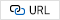8
Hwang I., Kim J., Choi H. S., Choi H., Lee J., Kim K. Y., Park J. B., Lee J. C., Ha J. B., Oh J. B., Shin J. K., Chung U. I., 2013, p-GaN gate HEMTs with tungsten gate metal for high threshold voltage and low gate current, IEEE Electron Device Letters, Vol. 34, No. 2, pp. 202-2049
Ge M., Ruzzarin M., Chen D., Lu H., Yu X., Zhou J., Santi C.D., Zhang R., Zheng Y., Meneghini M., Meneghesso G., Zanoni E., 2019, Gate reliability of p-GaN gate AlGaN/GaN high electron mobility transistors, IEEE Electron Device Letters, Vol. 40, No. 3, pp. 379-38210
Cai Y., Zhou Y., Lau K. M., Chen K. J., 2006, Control of threshold voltage of AlGaN/GaN HEMTs by fluoride-based plasma treatment: From depletion mode to enhancement mode, IEEE transactions on electron devices, Vol. 53, No. 9, pp. 2207-221511
Feng Z. H., Zhou R., Xie S. Y., Yin J. Y., Fang J. X., Liu B., Zhou W., Chen K.J., Cai S. J., 2010, 18-GHz 3.65-W/mm enhancement-mode AlGaN/GaN HFET using fluorine plasma ion implantation, IEEE electron device letters, Vol. 31, No. 12, pp. 1386-138812
Shen F., Hao R., Song L., Chen F., Yu G., Zhang X., Fan Y., Lin F., Cai Y., Zhang B., 2019, Enhancement mode AlGaN/GaN HEMTs by fluorine ion thermal diffusion with high V th stability, Applied Physics Express, Vol. 12, No. 6, pp. 06650113
Saito W., Takada Y., Kuraguchi M., Tsuda K., Omura I., 2006, Recessed-gate structure approach toward normally off high-voltage AlGaN/GaN HEMT for power electronics applications, IEEE Transactions on electron devices, Vol. 53, No. 2, pp. 356-36214
Oka T., Nozawa T., 2008, AlGaN/GaN recessed MIS-gate HFET with high-threshold-voltage normally-off operation for power electronics applications, 29, Vol. 7, pp. 668-67015
Kim K. W., Jung S. D., Kim D. S., Kang H. S., Im K. S., Oh J. J., Ha J.-B., Shin J.-K., Lee J. H., 2011, Effects of TMAH Treatment on Device Performance of Normally Off Al$_{2}$O$_{3}$/GaN MOSFET, IEEE electron device letters, Vol. 32, No. 10, pp. 1376-137816
Kim D. S., Im K. S., Kang H. S., Kim K. W., Bae S. B., Mun J. K., Nam E.-S., Lee J. H., 2012, Normally-off AlGaN/GaN metal–oxide–semiconductor heterostructure field-effect transistor with recessed gate and p-GaN back-barrier, Japanese Journal of Applied Physics, Vol. 51, No. 3R, pp. 03410117
Asubar J. T., Kawabata S., Tokuda H., Yamamoto A., Kuzuhara M., 2020, Enhancement-mode AlGaN/GaN MIS-HEMTs with high V$_{\mathrm{TH}}$ and high I$_{\mathrm{dmax}}$ using recessed-structure with regrown AlGaN barrier, IEEE Electron Device Letters, Vol. 41, No. 5, pp. 693-69618
Yagi S., Shimizu M., Inada M., Yamamoto Y., Piao G., Okumura H., Yano Y., Akutsu N., Ohashi H., 2006, High breakdown voltage AlGaN/GaN MIS-HEMT with SiN and TiO$_{2}$ gate insulator, Solid-State Electronics, Vol. 50, No. 6, pp. 1057-106119
Geng K., Chen D., Zhou Q., Wang H., 2018., AlGaN/GaN MIS-HEMT with PECVD SiNx, SiON, SiO$_{2}$ as gate dielectric and passivation layer, Electronics, Vol. 7, No. 12, pp. 41620
Jiang H., Liu C., Chen Y., Lu X., Tang C. W., Lau K. M., 2017, Investigation of in situ SiN as gate dielectric and surface passivation for GaN MISHEMTs, IEEE Transactions on Electron Devices, Vol. 64, No. 3, pp. 832-83921
Tang Z., Jiang Q., Lu Y., Huang S., Yang S., Tang X., Chen K. J., 2013, 600-V Normally Off SiN$_{\mathrm{x}}$/AlGaN/GaN MIS-HEMT With Large Gate Swing and Low Current Collapse, IEEE Electron Device Letters, Vol. 34, No. 11, pp. 1373-137522
Zhang Z., Yu G., Zhang X., Deng X., Li S., Fan Y., Sun S., Song L., Tan S., Wu D., Li W., Huang W., Fu K., Cai Y., Sun Q., Zhang B., 2016, Studies on high-voltage GaN-on-Si MIS-HEMTs using LPCVD Si$_{3}$N$_{4}$ as gate dielectric and passivation layer, IEEE Transactions on Electron Devices, Vol. 63, No. 2, pp. 731-73823
Arulkumaran S., Hong L. Z., Ing N. G., Selvaraj S. L., Egawa T., 2009., Influence of ammonia in the deposition process of SiN on the performance of SiN/AlGaN/GaN metal-insulator-semiconductor high-electron-mobility transistors on 4-in. Si (111), Applied Physics Express, Vol. 2, No. 3, pp. 03100124
Nicollian E. H., Goetzberger A., 1967, The si-sio, interface-electrical properties as determined by the metal-insulator-silicon conductance technique, The Bell System Technical Journal, Vol. 46, No. 6, pp. 1055-103325
Yang H., Son Y., Choi S., Hwang H., 2005, Improved conductance method for determining interface trap density of metal-oxide-semiconductor device with high series resistance, Japanese journal of applied physics, Vol. 44, No. 11L, pp. l1460Hee Dae An received the B.Sc. degree in School of Electronic Engineering, Kumoh National Insti-tute of Techology(KIT), Gumi, South Korea, in 2019, where he is currently pursuing the M.Sc. degree in School of Electronic and Electrical Engin-eering, Kyungpook National University (KNU), Daegu, South Korea. His research interests include the design, fabrication, and characterization of capacitor-less 1T-DRAM transistors and vertical GaN power devices.

So Ra Min received the B.Sc. degree in electronic engineering from the School of Electronics Engineering, Yeungnam University (YU), Gyeongsan, North Gyeongsang, South Korea, in 2020, and she is currently pursuing the M.Sc. degree with the School of Electronic and Electrical Engineering, Kyungpook National University (KNU), Daegu, South Korea. Her research interests include the design, fabrication, and characterization of GaN devices and capacitor-less 1T-DRAM transistors

Sang Ho Lee received the B.Sc. degree in electronics engineering from the School of Electronics Engineering (SEE), Kyungpook National University (KNU), Daegu, South Korea, in 2019, where he is currently pursuing the Ph.D. in School of Electronic and Electrical Engineering, Kyungpook National University (KNU), Daegu, South Korea. His research interests include the design, fabrication, and characterization of gate-all-around logic devices and capacitor-less 1T-DRAM transistors.

Jin Park received a B.Sc. degree in electronic engineering from the School of Electronics Engineering (SEE), Kyungpook National University (KNU), Daegu, South Korea, in 2020, where she is currently pursuing the M.Sc. degree in School of Electronic and Electrical Engineering, Kyungpook National University (KNU), Daegu, South Korea. Her research interests include the design, fabrication, and characterization of gate-all-around logic devices and capacitor-less 1T-DRAM transistors.

Geon Uk Kim received a B.Sc. degree in electronic engineering from the School of Electronics Engi-neering (SEE), Kyungpook National University (KNU), Daegu, South Korea, in 2021, where he is currently pursuing the M.Sc. degree in School of Electronic and Electrical Engineering, Kyungpook National University (KNU), Daegu, South Korea. His research interests include the design, fabrication, and characterization of GaN devices and capacitor-less 1T-DRAM transistors.

Young Jun Yoon received the B.S. and Ph.D. degrees in electronics engineering from Kyungpook Natio-nal University, Daegu, Korea, in 2013 and 2019, respectively. He is currently postdoctoral researcher wi-th Korea Multi-purpose Accelerator Complex, Korea Atomic Energy Research Institute (KAERI). His research interests include design, fabrication, and characterization of logic transistor and memory.

Jae Hwa Seo received the B.S. and Ph.D. degree in Electronics Engin-eering from the School of Electronics Engineering, Kyungpook National University (KNU), Daegu, Korea, in 2012, 2018. He worked as a Post Doc. in electrical engineering from School of Electrical Engineering and Computer Science (EECS), Seoul National University (SNU), Seoul, Korea, in 2018 to 2019. Now, he has worked as reseacher at Power Semiconductor Research Center, Korea Electro-technology Research Institute. His research interests include the design, fabrication and characterization of V-NAND/1T-DRAM devices, nano-scale CMOS, tunneling FETs, and compound/silicon-based transistors.

Min Su Cho received a B.Sc. degree in computer engineering from the College of Electrical and Computer Engineering, Chungbuk National University (CBNU), Cheongju, South Korea, in 2015, and an M.Sc. degree from the School of Electronics Engineering (SEE), Kyungpook National University (KNU), and Ph.D. degree in Electronics Engineering from the School of Electronic and Electrical Engineering. He has worked as researcher at DB HiTek. His research interests include the design, fabrication, and characterization of compound CMOS, tunneling FETs, and III–V compound transistors.

Jae Won Jang received the B.S. and M.S degrees in electrical engineering from Korea University, Seoul, Korea in 2006 and 2008, respectively. In 2013, Jaewon Jang received Ph.D degrees in electrical engineering and computer sciences from University of California at Berkeley, CA, USA. From 2013 to 2014, he was a post doctorial researcher, and working for developing of high-performance metal oxide transistors by printing technology. From 2015 to 2016, he was a researcher and working for developing of high performance organic thin film transistor in Samsung Advanced Institute and Technology, Suwon, Korea. Since 2016, he has been with Kyungpook National University, Daegu, Korea, where he is currently an assistant professor with the School of Electronics Engineering.

Jin-Hyuk Bae received a B.S. degree in Electronics and Electrical Engin-eering from Kyungpook Na-tional University, Daegu, Korea in 2004, and M.S. and Ph.D. degrees in Electrical Engineering from the Seoul National University, Seoul, Korea in 2006 and 2010, respectively. For the period from 2010 to 2012, he worked as a postdoctoral research fellow with Ecole Nationale Superiere des Mines de Saint-Etienne, Gardanne, France. In 2012, he joined the faculty in the School of Electronics Engineering, Kyungpook National University, Korea, where he is currently an Associate Professor. His research interests include interfacial engineering and physics of organic based and metal-oxide-based electronic devices and their sensor applications.

Sin-Hyung Lee received his B.S. and Ph.D. degrees in electrical engin-eering from Seoul National Univer-sity, Korea in 2013 and 2019, respectively. He is currently an assistant professor in the School of Electronics Engineering at Kyung-pook National University in Republic of Korea. His research covers the neuromorphic electronics, artificial synapse, memristors, and organic electronics.

In Man Kang received the B.S. degree in electronic and electrical engineering from School of Elec-tronics and Electrical Engineering, Kyungpook National University (KNU), Daegu, Korea, in 2001, and the Ph.D. degree in electrical engin-eering from School of Electrical Engineering and Computer Science (EECS), Seoul National University (SNU), Seoul, Korea, in 2007. He worked as a teaching assistant for semiconductor process education from 2001 to 2006 at Inter-university Semiconductor Research Center (ISRC) in SNU. From 2007 to 2010, he worked as a senior engineer at Design Technology Team of Samsung Electronics Company. In 2010, he joined KNU as a full-time lecturer of the School of Electronics Engineering (SEE). Now, he is currently working as an associate professor. His current research interests include CMOS RF modeling, silicon nanowire devices, tunneling transistor, low-power nano CMOS, and III-V compound semiconductors. He is a member of IEEE EDS.